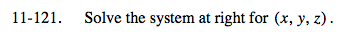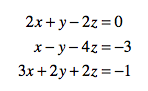### Home > A2C > Chapter Ch11 > Lesson 11.2.6 > Problem11-121

11-121.(a) 2x + y − 2z = 0
xy − 4z = −3
3x − 6z = −3

2(xy − 4z) → 2x − 2y − 8z = −6(b) 3x + 2y + 2z = −1
5x − 6z = −7

Now use equations (a) and (b) to solve for x and z, and use those values to solve for y.

$\left(-2, 3, -\frac{1}{2}\right)$